# Time and Space Complexity

## Time and Space Complexity:

In this article, I am going to discuss Time and Space Complexity with Examples. Please read our previous article where we discussed Abstract Data Type (ADT) in detail. Time and Space Complexity is a very important topic and sometimes it is difficult for the students to understand even though it is not that difficult. It is not that complex even though the word complexity is used, it is very simple. First, we will learn about time complexity and then we will learn about space complexity.

##### Time Complexity:

In daily life when we do any work or any task, we want to know how much time it takes for performing that particular task. For example, it is a one-hour task or a one-day task, that amount of time required depending on the work that we have to do. So, usually in our daily life, we measure the time based on the work that we have to do.

Nowadays, we are using machines to do our work i.e. computers to do our work. We want to know how much time the machine takes for doing the same task. For example, if a person is making one bread in 15-20 minutes, then if we are using a machine for making that bread, then how much time the machine takes? We are interested in that one. If the machine is taking 4-5 hours then it is better to do it manually.

So, how much time a machine takes is very important for us. We use computers for problem-solving. What type of problem-solving? The work that we used to do using pen and paper, that same work we want our computer to do.

So, computers are used for performing computation tasks and computation also needs time. We want to measure how much time a machine will take. Actually, that depends on the process or the procedure for completing that task. The time complexity basically depends on the procedure that we are adopting. Now we are going to discuss variable examples and will see what procedure takes what amount of time.

##### Array:

Let us start with the array. The following is an array with some size and some elements are there. The example shown below has 10 elements. But the question is will it be always 10 elements or depends on the problem? The answer is depending on the problem. So, how many elements are there in the array, let say n number of elements. We don’t know how many elements will be there maybe 10, 10000, or 10000000. We don’t know, so we can say n elements (n means some number of elements). That number of elements may start from 1 and goes up to infinity. We don’t define infinity, so we can say up to the maximum number or whatever that number we can imagine we take that one.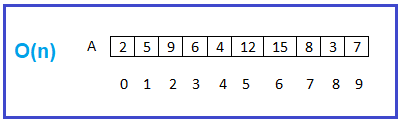Now what we want to do with all elements? We want to add all of them and so we have to go through all of them one by one and adding them. So how much time it will take? It depends on the number of elements. As there is n number of elements, so the time taken will be n.

The next thing we want to do is we want to search for a particular number i.e. whether element 12 is there or not. Search for it, and it is there in the list. Again, we want to search for element 21, is it there or not? Check for all, No, 21 is not there. At most, how much time is it taking? It depends on the number of elements that we have to compare. So how many elements are there, n elements are there. So, what is the time taken? N time is taken.

It means in a list if you have some n elements and if you are going through all of them just once, then the time is n and we represent this n as a degree. So, we can say order(n) i.e. O(n). Usually use the term degree and order are the same thing). There are other terms like O, Ω, Ɵ that we will discuss at the end of the course because till then you will be having a good understanding of time complexities. So, throughout this course, we’ll be using term order. When we know what are asymptotic notations, like O, Ω, Ɵ then we will understand how to use them.

The next very important thing, if you want to access all the elements of the list i.e. from the array, what is the code that you have to write? You have to write a for loop as shown below. Within the body whatever you want to do, you can. For example, you can write the code for search something or add all of them or count the number of elements, or finding the maximum element. So, whatever you want to do that logic or procedure comes there.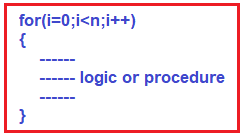The above for loop is taking us through all those elements. As there is n number of elements so what is the time? It is order(n). For finding the time complexity either you can measure the time based on the work that you are doing or else from the program code you can also find the time complexity. If there is a loop going through 0 to the last element means it is n i.e. it is taking an order(n) time. Whatever it is there inside the for loop, it will repeat the same for n times. So, we can analyze the time based on the procedure as well as based on the code.

The most confusing thing is when the code is given, we get confused about how to analyze this one. So, actually, what the code is doing, you do that work and based on the work you analyze it. It’s very simple. If you don’t want to understand what the code is doing, then it is a very difficult task. So, if a for loop is used means there is a chance that time is order(n).

##### Next Situation:

Now, let us move to the next situation. In that list, I want to compare each element with all other elements. For example, for the 1st element we are comparing or processing with all other elements, and again for the second element, we are processing it with all other elements and so on. This type of processing may be required when we are comparing for sorting purposes.

In this case, n elements are being processed but how many times? For each element n elements are processed, this will be n2. We can say order(n2). Now, in the same case, we will see one more thing. For processing like this, how the code should look like? The code should have a nested for loop as shown below.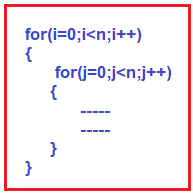So, when we have two nested ‘for’ loops, it is n2.

##### The third situation for the same array:

Suppose being on the first element, we want to process the rest of the elements. i.e. n-1 elements. Being on the second element I am processing the rest of the element but not the first element i.e. n-2 element. Similarly, being on the third element we are processing the rest of the elements but not with the 1st and 2nd element i.e. n-3 elements, and so on. For better understanding, please have a look at the below code.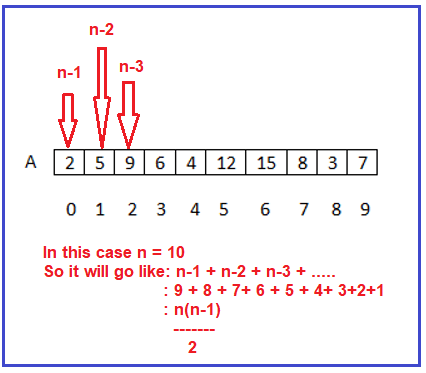So, 1+2+3+4+…. +n-3+n-2+n-1

=n(n-1)/2

=(n2-n)2

So, the degree of this polynomial is n2 i.e. O(n2). Let us see how to write the same thing using code as shown below.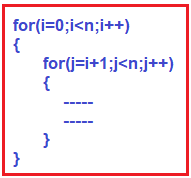##### Fourth Situation:

Suppose first of all we are processing the middle element. Then again either on the left or on the right side of the middle element, we process the middle element and so on. In this case, we are not processing the entire list, we are processing half of the list, again half of the list, and again half of the list and that process is always dividing the list by two. For better understanding, please have a look at the below image.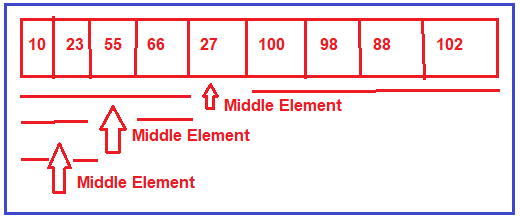When something is successively divided until it reaches one, that is represented as log and we are dividing it 2 and the number of elements is n log of n elements. So, the time complexity is log2n. The code for the above cases is given below.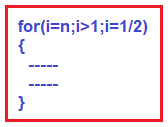##### Matrix:

Matrix is having how many elements. The matrix showing in the below image is a 4*4 dimensions matrix. Total how many elements? If dimensions are n*n then total n2 elements.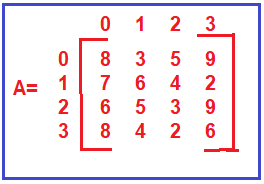When we are processing upon a matrix then it will require n2 amount of time if we are processing all the elements. If we say we are just processing a row then a row is having n elements so the order is O(n). Similarly, if we are processing a column again it is having n elements so the time will be O(n). If we are processing all elements then it is n2. Now, we will show the code for processing a matrix of elements. We need two nested ‘for’ loops as shown below.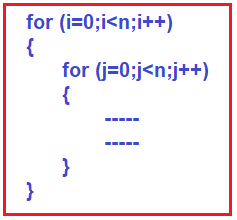In the next article, I am going to give you a brief introduction to Algorithms. Here, in this article, I try to explain Time and Space Complexity and I hope you enjoy this Time and Space Complexity article. I would like to have your feedback. Please post your feedback, question, or comments about this Time and Space Complexity article.

### 2 thoughts on “Time and Space Complexity”

1.2.